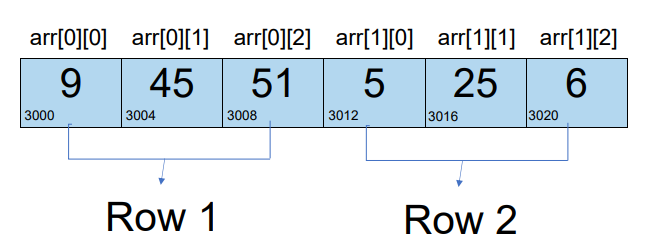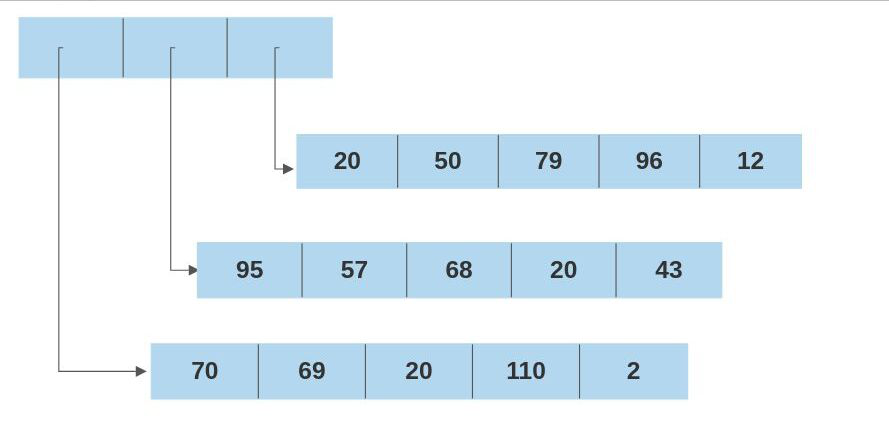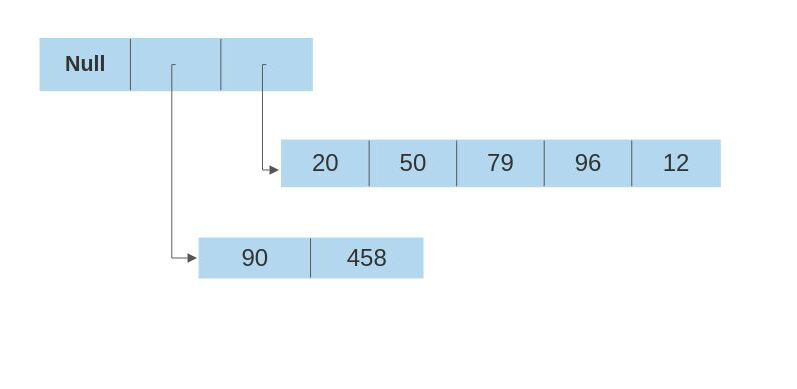# Difference between multidimensional array in C++ and Java

• Difficulty Level : Easy
• Last Updated : 02 Sep, 2021

Prerequisites: Multidimensional array in C++, Multidimensional array in Java

### Multidimensional Arrays:

Multidimensional arrays are a tabular representation of arrays to store multiple elements. These dimensions can be 1D arrays, 2D-arrays, etc. Multidimensional arrays are available in both C++ and Java, but their implementation and some properties are different.

Want to learn from the best curated videos and practice problems, check out the C++ Foundation Course for Basic to Advanced C++ and C++ STL Course for the language and STL. To complete your preparation from learning a language to DS Algo and many more, please refer Complete Interview Preparation Course.

## Implementation in C/C++ :

In C++ a multidimensional array is created internally as a giant linear array. C++ syntax abstracts this linear block of memory into a 2 or 3-dimensional behavior making it easy for the programmer.

Examples:

A 2D array of dimensions 2 rows x 3 cols {{9, 45, 51}, {5, 25, 6}} is implemented as follows (Assuming Integer takes 4 bytes):Hence, the inner formula for inner element at particular index is given as:

arr[rowIndex][colIndex] = arr + (rowIndex * noOfCols * sizeOfDataType) + coLIndex * sizeOfDataType

Let assume base address to be 3000. Then arr = 3000 + (1 * 3 * 4) + 1 * 4 = 3000 + 12 + 4 = 3016.

Because of such implementation, the number of columns must be equal for each row, and it is mandatory to specify column size while declaration in order to access elements properly.

Below is the implementation of the multidimensional array in C++:

## C++

 `// C++ program for multidimention array``// implementation``#include ``using` `namespace` `std;` `// Driver Code``int` `main()``{``    ``// Create a 2d integer array,``    ``// dimensions: 3rows X 5cols``    ``int` `arr = {``        ``{ 23, 56, 34, 52, 63 },``        ``{ 40, 20, 96, 43, 97 },``        ``{ 75, 51, 10, 82, 43 }``    ``};` `    ``// Traversing of 2D array``    ``cout << ``"Printing entire 2d array: "``         ``<< endl;` `    ``// Iterate over the rows``    ``for` `(``int` `i = 0; i < 3; i++) {` `        ``// Iterate over the cols``        ``for` `(``int` `j = 0; j < 5; j++) {` `            ``cout << ``"arr["` `<< i << ``"]["` `<< j``                 ``<< ``"]:"` `<< arr[i][j]``                 ``<< ``"      "``;``        ``}``        ``cout << endl;``    ``}` `    ``return` `0;``}`
Output:

```Printing entire 2d array:
arr:23      arr:56      arr:34      arr:52      arr:63
arr:40      arr:20      arr:96      arr:43      arr:97
arr:75      arr:51      arr:10      arr:82      arr:43```

### Implementation in Java:

In Java, a multidimensional array is implemented as an array of arrays where each index of the base array refers to an entirely different array. So, arr[rowIndex] returns an entire single dimensional array and arr[rowIndex][coLIndex] returns the element at index coLIndex in that single dimensional array.

Examples:

A 2D array of dimensions 3 rows x 5 cols is implemented as follows:Because of this structure, It is possible to have 2D arrays with different column sizes (even null values) in Java.Below is the implementation of the multidimensional array in Java:

## Java

 `// Java program for multidimensional``// array implementation``import` `java.io.*;` `class` `GFG {` `    ``// Driver Code``    ``public` `static` `void` `main(String[] args)``    ``{` `        ``// Create a 2D integer array``        ``// dimensions: 3rows X 5cols``        ``int``[][] arr = {``            ``{ ``23``, ``56``, ``34``, ``52``, ``63` `},``            ``{ ``40``, ``20``, ``96``, ``43``, ``97` `},``            ``{ ``75``, ``51``, ``10``, ``82``, ``43` `}``        ``};` `        ``// Traversing the 2D array``        ``System.out.println(``"Printing entire 2d array: "``);` `        ``// Iterate over the rows``        ``for` `(``int` `i = ``0``;``             ``i < arr.length; i++) {` `            ``// Iterate over the cols``            ``for` `(``int` `j = ``0``;``                 ``j < arr[i].length; j++) {``                ``System.out.print(``                    ``"arr["` `+ i + ``"]["` `+ j``                    ``+ ``"]:"` `+ arr[i][j]``                    ``+ ``"    "``);``            ``}``            ``System.out.println();``        ``}``        ``System.out.println();` `        ``// Reassigning arr to another``        ``// array` `        ``// This is not possible in 2D``        ``// arrays in C++, instead of``        ``// there is array of pointers``        ``arr[``2``] = ``new` `int``[] { ``82``, ``53``, ``64``,``                             ``12``, ``45``, ``3` `};` `        ``// Traversing the array again``        ``System.out.println(``            ``"Printing entire 2d array "``            ``+ ``"after modification: "``);` `        ``// Iterate over the rows``        ``for` `(``int` `i = ``0``;``             ``i < arr.length; i++) {` `            ``// Iterate over the cols``            ``for` `(``int` `j = ``0``;``                 ``j < arr[i].length; j++) {` `                ``System.out.print(``                    ``"arr["` `+ i + ``"]["` `+ j``                    ``+ ``"]:"` `+ arr[i][j]``                    ``+ ``"    "``);``            ``}``            ``System.out.println();``        ``}``    ``}``}`
Output
```Printing entire 2d array:
arr:23    arr:56    arr:34    arr:52    arr:63
arr:40    arr:20    arr:96    arr:43    arr:97
arr:75    arr:51    arr:10    arr:82    arr:43

Printing entire 2d array after modification:
arr:23    arr:56    arr:34    arr:52    arr:63
arr:40    arr:20    arr:96    arr:43    arr:97
arr:82    arr:53    arr:64    arr:12    arr:45    arr:3    ```

My Personal Notes arrow_drop_up